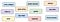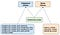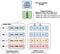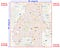# Demonstration of TensorFlow Feature Columns (tf.feature_column)

In this tutorial you will learn:

1. What is TensorFlow Feature Columns

# Feature Columns

This tutorial details feature columns. Think of feature columns as the intermediaries between raw data and Estimators. Feature columns are very rich, enabling you to transform a diverse range of raw data into formats that Estimators can use, allowing easy experimentation.

In simple words feature column are bridge between raw data and estimator or model.Some real-world features (such as, longitude) are numerical, but many are not.

Input to a Deep Neural Network

What kind of data can a deep neural network operate on? The answer is, of course, numbers (for example, tf.float32). After all, every neuron in a neural network performs multiplication and addition operations on weights and input data. Real-life input data, however, often contains non-numerical (categorical) data. For example, consider a product_class feature that can contain the following three non-numerical values:

• kitchenware

ML models generally represent categorical values as simple vectors in which a 1 represents the presence of a value and a 0 represents the absence of a value. For example, when product_class is set to sports, an ML model would usually represent product_class as [0, 0, 1], meaning:

• 0: kitchenware is absent

So, although raw data can be numerical or categorical, an ML model represents all features as numbers.

# Feature Columns

As the following figure suggests, you specify the input to a model through the feature_columns argument of an Estimator (DNNClassifier for Iris). Feature Columns bridge input data (as returned by input_fn) with your model.

To create feature columns, call functions from the tf.feature_column module. This tutorial explains nine of the functions in that module. As the following figure shows, all nine functions return either a Categorical-Column or a Dense-Column object, except bucketized_column, which inherits from both classes:Feature column methods fall into two main categories and one hybrid category.

Let’s look at these functions in more detail.

# Import TensorFlow and other libraries

`from __future__ import absolute_import, division, print_function, unicode_literalsimport numpy as npimport pandas as pdimport tensorflow as tffrom tensorflow import feature_columnfrom tensorflow.keras import layers`

# Create Demo data

`data = {'marks': [55,21,63,88,74,54,95,41,84,52],        'grade': ['average','poor','average','good','good','average','good','average','good','average'],        'point': ['c','f','c+','b+','b','c','a','d+','b+','c']}`

# Demo Data

`df = pd.DataFrame(data)df`

# Demonstrate several types of feature column

`# A utility method to show transromation from feature columndef demo(feature_column):  feature_layer = layers.DenseFeatures(feature_column)  print(feature_layer(data).numpy())`

# Numeric columns

The output of a feature column becomes the input to the model (using the demo function defined above, we will be able to see exactly how each column from the dataframe is transformed). A numeric column is the simplest type of column. It is used to represent real valued features. When using this column, your model will receive the column value from the dataframe unchanged.

`marks = feature_column.numeric_column("marks")demo(marks)>> [[55.]   [21.]   [63.]   [88.]   [74.]   [54.]   [95.]   [41.]   [84.]   [52.]]`

# Bucketized columns

Often, you don’t want to feed a number directly into the model, but instead split its value into different categories based on numerical ranges. Consider raw data that represents a person’s age. Instead of representing age as a numeric column, we could split the age into several buckets using a bucketized column. Notice the one-hot values below describe which age range each row matches. Buckets include the left boundary, and exclude the right boundary. For example, consider raw data that represents the year a house was built. Instead of representing that year as a scalar numeric column, we could split the year into the following four buckets:

The model will represent the buckets as follows:

Why would you want to split a number — a perfectly valid input to your model — into a categorical value? Well, notice that the categorization splits a single input number into a four-element vector. Therefore, the model now can learn four individual weights rather than just one; four weights creates a richer model than one weight. More importantly, bucketizing enables the model to clearly distinguish between different year categories since only one of the elements is set (1) and the other three elements are cleared (0). For example, when we just use a single number (a year) as input, a linear model can only learn a linear relationship. So, bucketing provides the model with additional flexibility that the model can use to learn.

The following code demonstrates how to create a bucketized feature:

`marks_buckets = feature_column.bucketized_column(marks, boundaries=[30,40,50,60,70,80,90])demo(marks_buckets)>> [[0. 0. 0. 1. 0. 0. 0. 0.]   [1. 0. 0. 0. 0. 0. 0. 0.]   [0. 0. 0. 0. 1. 0. 0. 0.]   [0. 0. 0. 0. 0. 0. 1. 0.]   [0. 0. 0. 0. 0. 1. 0. 0.]   [0. 0. 0. 1. 0. 0. 0. 0.]   [0. 0. 0. 0. 0. 0. 0. 1.]   [0. 0. 1. 0. 0. 0. 0. 0.]   [0. 0. 0. 0. 0. 0. 1. 0.]   [0. 0. 0. 1. 0. 0. 0. 0.]]`

# Indicator and embedding columns

Indicator columns and embedding columns never work on features directly, but instead take categorical columns as input.

## Indicator columns

In this dataset, grade is represented as a string (e.g. ‘poor’, ‘average’, or ‘good’). We cannot feed strings directly to a model. Instead, we must first map them to numeric values. The categorical vocabulary columns provide a way to represent strings as a one-hot vector (much like you have seen above with age buckets). The vocabulary can be passed as a list using categorical_column_with_vocabulary_list, or loaded from a file using categorical_column_with_vocabulary_file.

We cannot input strings directly to a model. Instead, we must first map strings to numeric or categorical values. Categorical vocabulary columns provide a good way to represent strings as a one-hot vector. For example:

`grade = feature_column.categorical_column_with_vocabulary_list(      'grade', ['poor', 'average', 'good'])grade_one_hot = feature_column.indicator_column(grade)demo(grade_one_hot)>> [[0. 1. 0.]   [1. 0. 0.]   [0. 1. 0.]   [0. 0. 1.]   [0. 0. 1.]   [0. 1. 0.]   [0. 0. 1.]   [0. 1. 0.]   [0. 0. 1.]   [0. 1. 0.]]`

# Embedding columns

Suppose instead of having just a few possible strings, we have thousands (or more) values per category. For a number of reasons, as the number of categories grow large, it becomes infeasible to train a neural network using one-hot encodings. We can use an embedding column to overcome this limitation. Instead of representing the data as a one-hot vector of many dimensions, an embedding column represents that data as a lower-dimensional, dense vector in which each cell can contain any number, not just 0 or 1. The size of the embedding (8, in the example below) is a parameter that must be tuned.

Key point: using an embedding column is best when a categorical column has many possible values. We are using one here for demonstration purposes, so you have a complete example you can modify for a different dataset in the future.

## Point column as indicator_column

`point = feature_column.categorical_column_with_vocabulary_list( ‘point’, df[‘point’].unique())point_one_hot = feature_column.indicator_column(point)demo(point_one_hot)>> [[1. 0. 0. 0. 0. 0. 0.]   [0. 1. 0. 0. 0. 0. 0.]   [0. 0. 1. 0. 0. 0. 0.]   [0. 0. 0. 1. 0. 0. 0.]   [0. 0. 0. 0. 1. 0. 0.]   [1. 0. 0. 0. 0. 0. 0.]   [0. 0. 0. 0. 0. 1. 0.]   [0. 0. 0. 0. 0. 0. 1.]   [0. 0. 0. 1. 0. 0. 0.]   [1. 0. 0. 0. 0. 0. 0.]]`

## Point column as embedding_column

`# Notice the input to the embedding column is the categorical column# we previously createdpoint_embedding = feature_column.embedding_column(point, dimension=4)demo(point_embedding)>>[[ 0.6905385  -0.08270663  0.15046535 -0.3439266 ]   [ 0.56737125  0.06695139 -0.58371276  0.49233127]   [ 0.4538004  -0.03839593 -0.4058998  -0.1113084 ]   [-0.50984156 -0.11315092  0.39700866  0.09811988]   [ 0.35654882  0.41658938 -0.67096025 -0.2758388 ]   [ 0.6905385  -0.08270663  0.15046535 -0.3439266 ]   [ 0.58311635  0.6259656  -0.27828178  0.14894487]   [-0.721768   -0.0898371   0.5906883  -0.4207294 ]   [-0.50984156 -0.11315092  0.39700866  0.09811988]   [ 0.6905385  -0.08270663  0.15046535 -0.3439266 ]]`

When using an indicator column, we’re telling TensorFlow to do exactly what we’ve seen in our categorical product_class example. That is, an indicator column treats each category as an element in a one-hot vector, where the matching category has value 1 and the rest have 0s:

Now, suppose instead of having just three possible classes, we have a million. Or maybe a billion. For a number of reasons, as the number of categories grow large, it becomes infeasible to train a neural network using indicator columns.

We can use an embedding column to overcome this limitation. Instead of representing the data as a one-hot vector of many dimensions, an embedding column represents that data as a lower-dimensional, ordinary vector in which each cell can contain any number, not just 0 or 1. By permitting a richer palette of numbers for every cell, an embedding column contains far fewer cells than an indicator column.

Let’s look at an example comparing indicator and embedding columns. Suppose our input examples consist of different words from a limited palette of only 81 words. Further suppose that the data set provides the following input words in 4 separate examples:

• “dog”

In that case, the following figure illustrates the processing path for embedding columns or indicator columns.An embedding column stores categorical data in a lower-dimensional vector than an indicator column. (We just placed random numbers into the embedding vectors; training determines the actual numbers.)

When an example is processed, one of the categorical_column_with… functions maps the example string to a numerical categorical value. For example, a function maps “spoon” to . (The 32 comes from our imagination — the actual values depend on the mapping function.) You may then represent these numerical categorical values in either of the following two ways:

• As an indicator column. A function converts each numeric categorical value into an 81-element vector (because our palette consists of 81 words), placing a 1 in the index of the categorical value (0, 32, 79, 80) and a 0 in all the other positions.

How do the values in the embeddings vectors magically get assigned? Actually, the assignments happen during training. That is, the model learns the best way to map your input numeric categorical values to the embeddings vector value in order to solve your problem. Embedding columns increase your model’s capabilities, since an embeddings vector learns new relationships between categories from the training data.

# Hashed feature columns

Another way to represent a categorical column with a large number of values is to use a categorical_column_with_hash_bucket. This feature column calculates a hash value of the input, then selects one of the `hash_bucket_size` buckets to encode a string. When using this column, you do not need to provide the vocabulary, and you can choose to make the number of hash_buckets significantly smaller than the number of actual categories to save space.

Key point: An important downside of this technique is that there may be collisions in which different strings are mapped to the same bucket. In practice, this can work well for some datasets regardless.

`point_hashed = feature_column.categorical_column_with_hash_bucket(      'point', hash_bucket_size=4)demo(feature_column.indicator_column(point_hashed))>> [[1. 0. 0. 0.] [1. 0. 0. 0.] [0. 1. 0. 0.] [0. 0. 0. 1.] [0. 0. 1. 0.] [1. 0. 0. 0.] [0. 0. 0. 1.] [1. 0. 0. 0.] [0. 0. 0. 1.] [1. 0. 0. 0.]]`

At this point, you might rightfully think: “This is crazy!” After all, we are forcing the different input values to a smaller set of categories. This means that two probably unrelated inputs will be mapped to the same category, and consequently mean the same thing to the neural network. The following figure illustrates this dilemma, showing that kitchenware and sports both get assigned to category (hash bucket) 12:

As with many counter-intuitive phenomena in machine learning, it turns out that hashing often works well in practice. That’s because hash categories provide the model with some separation. The model can use additional features to further separate kitchenware from sports.

# Crossed feature columns

Combining features into a single feature, better known as feature crosses, enables a model to learn separate weights for each combination of features. Here, we will create a new feature that is the cross of marks and age. Note that `crossed_column` does not build the full table of all possible combinations (which could be very large). Instead, it is backed by a `hashed_column`, so you can choose how large the table is.

Combining features into a single feature, better known as feature crosses, enables the model to learn separate weights for each combination of features.

More concretely, suppose we want our model to calculate real estate prices in Atlanta, GA. Real-estate prices within this city vary greatly depending on location. Representing latitude and longitude as separate features isn’t very useful in identifying real-estate location dependencies; however, crossing latitude and longitude into a single feature can pinpoint locations. Suppose we represent Atlanta as a grid of 100x100 rectangular sections, identifying each of the 10,000 sections by a feature cross of latitude and longitude. This feature cross enables the model to train on pricing conditions related to each individual section, which is a much stronger signal than latitude and longitude alone.

The following figure shows our plan, with the latitude & longitude values for the corners of the city in red text:Map of Atlanta. Imagine this map divided into 10,000 sections of equal size.
`crossed_feature = feature_column.crossed_column([marks_buckets, grade], hash_bucket_size=10)demo(feature_column.indicator_column(crossed_feature))>>[[0. 0. 0. 0. 0. 0. 0. 0. 1. 0.] [0. 0. 0. 0. 1. 0. 0. 0. 0. 0.] [0. 0. 0. 0. 1. 0. 0. 0. 0. 0.] [0. 0. 0. 0. 1. 0. 0. 0. 0. 0.] [1. 0. 0. 0. 0. 0. 0. 0. 0. 0.] [0. 0. 0. 0. 0. 0. 0. 0. 1. 0.] [0. 0. 0. 0. 0. 1. 0. 0. 0. 0.] [0. 0. 0. 0. 0. 0. 1. 0. 0. 0.] [0. 0. 0. 0. 1. 0. 0. 0. 0. 0.] [0. 0. 0. 0. 0. 0. 0. 0. 1. 0.]]`

You may create a feature cross from either of the following:

• Feature names; that is, names from the dict returned from input_fn.

Except that a full grid would only be tractable for inputs with limited vocabularies. Instead of building this, potentially huge, table of inputs, the crossed_column only builds the number requested by the hash_bucket_size argument. The feature column assigns an example to a index by running a hash function on the tuple of inputs, followed by a modulo operation with hash_bucket_size.

As discussed earlier, performing the hash and modulo function limits the number of categories, but can cause category collisions; that is, multiple (latitude, longitude) feature crosses will end up in the same hash bucket. In practice though, performing feature crosses still adds significant value to the learning capability of your models.

Somewhat counterintuitively, when creating feature crosses, you typically still should include the original (uncrossed) features in your model (as in the preceding code snippet). The independent latitude and longitude features help the model distinguish between examples where a hash collision has occurred in the crossed feature.

## How to use Feature Columns in tf.keras models

Find this tutorial here

## How to use Feature Columns in tf.estimator models (linear and tree based model)

Find this tutorial here